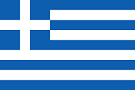Greece National Olympiad Problem 4

Algebra Level 5Let $a,b,c$ be positive real numbers with sum $6$. Find the maximum value of $S = \sqrt{{{a^2} + 2bc}} + \sqrt{{{b^2} + 2ca}} + \sqrt{{{c^2} + 2ab}}.$

This problem is part of this set.

×